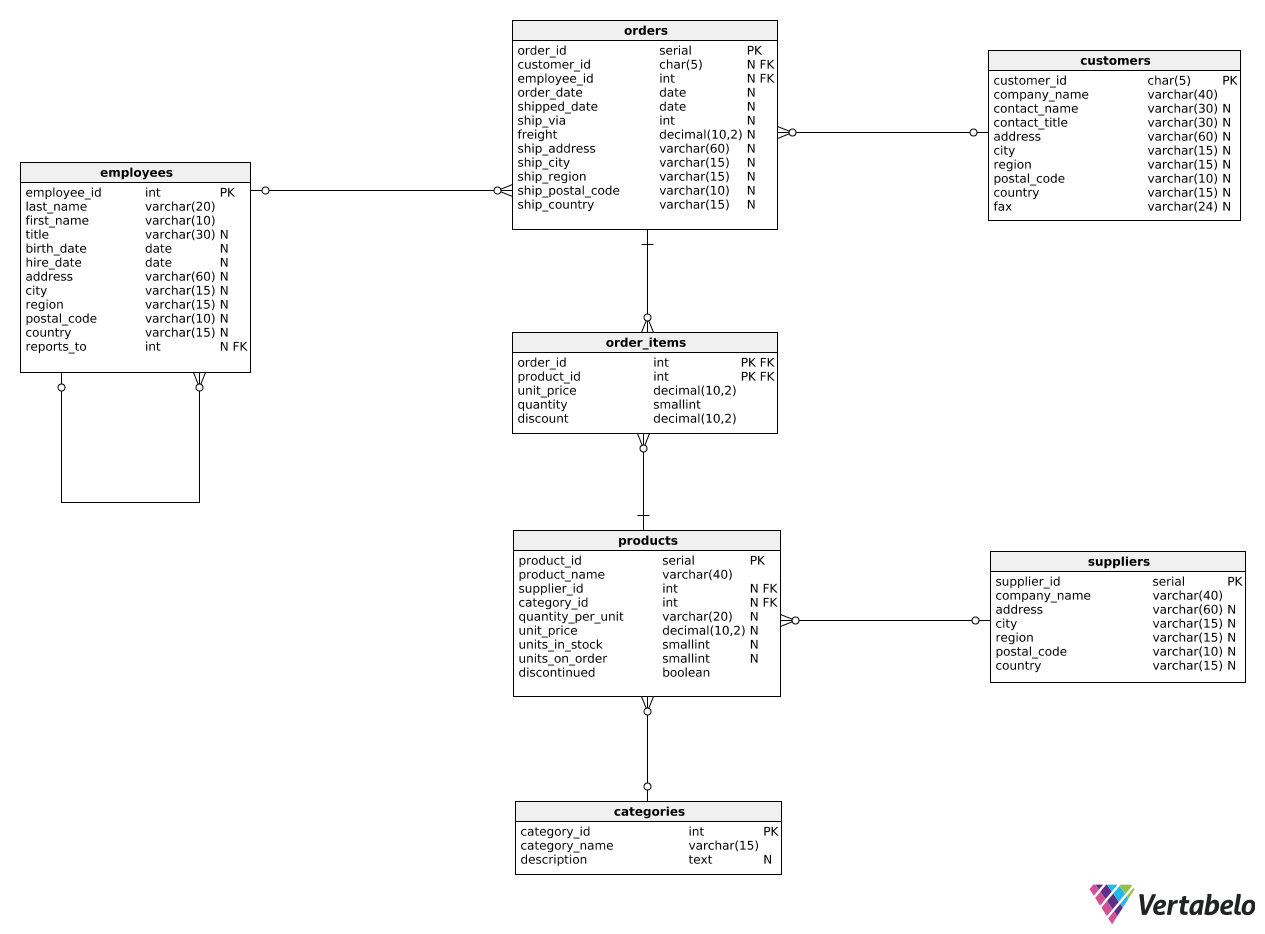Deals Of The Week - hours only!Up to 80% off on all courses and bundles.-Close
Introduction
Basic multi-level aggregation
Multi-level aggregation in groups
Multi-level aggregation with custom classification
Three or more aggregation levels
13. Three aggregation levels – example 1
Summary

## Instruction

Very well done!

Sometimes, we need more than two aggregation levels. For instance, we could calculate the average order value for each customer and then find the maximum average. Take a look at the query below:

WITH order_values AS (
SELECT
customer_id,
SUM(unit_price * quantity) AS total_price
FROM orders o
JOIN order_items oi
ON o.order_id = oi.order_id
GROUP BY customer_id
),

customer_averages AS (
SELECT
customer_id,
AVG(total_price) AS avg_total_price
FROM order_values
GROUP BY customer_id
)

SELECT
MAX(avg_total_price) AS maximal_average
FROM customer_averages;


Notice that we introduced two CTEs this time. There is no WITH keyword before the second CTE; we simply need a comma to separate the two.

In the first CTE, we calculate the value of each order and select it alongside customer_id. In the second CTE, we compute the average order value for each customer based on the first CTE. Finally, in the outer query, we find the maximum average value from the second CTE.

## Exercise

For each employee, calculate the average order value (after discount) and then show the minimum average (name the column minimal_average) and the maximum average (name the column maximal_average) values.

Hint: Use two CTEs. In the first CTE, select the employee_id and the total price after discount for each order. In the second CTE, calculate the average order value after discount for each employee. Finally, in the outer query, use the MIN() and MAX() functions.

### Stuck? Here's a hint!

Use the following template:

WITH order_values AS (
...
),
customer_averages AS (
...
)
SELECT
MIN(...) AS minimal_average,
MAX(...) AS maximal_average
FROM customer_averages;